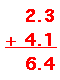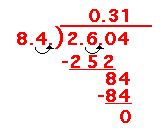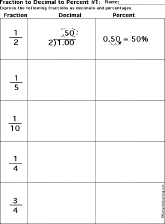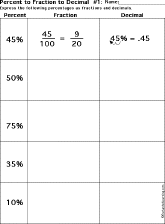EnchantedLearning.com is a user-supported site.
As a bonus, site members have access to a banner-ad-free version of the site, with print-friendly pages.

 More MathRounding EnchantedLearning.comDecimals Fractions
 PlaceValue AddingDecimals SubtractingDecimals MultiplyingDecimals DividingDecimals Worksheetsto PrintA decimal is a number that is written with a decimal point in it. For example, the numbers 4.1, 12.34, and 0.013 are decimals. The value of the digits is based on the number ten.

The decimal numeration system is based on base 10. Each position in a number has a value that is a power of 10. A decimal point separates the non-negative powers of 10 (100=1, 101=10, 102=100, 103=1,000, etc.) on the left from the negative exponents (10-1=1/10, 10-2=1/100, 10-3=1/1000, etc.) on the right.

Decimal Number Written as a Fraction Written in Terms of Place Value
4.1 4 1/10 4 plus 1 tenth
12.34 12 34/100 1 ten plus 2 ones plus 3 tenths and 4 hundredths
0.01 1/100 1 hundredth

The following chart shows the value of each place in a decimal number (written as a simple number, as an exponent, and in English):

100,000 = 105
hundred thousands
10,000 = 104
ten thousands
1,000 = 103
thousands
100 = 102
hundreds
10 = 101
tens
1 = 100
ones
decimal point 1/10 = 10-1
tenths
1/100 = 10-2
hundredths
1/1,000 = 10-3
thousandths
1/10,000 = 104
ten thousandths
6 5 4 3 2 1 . 1 2 3 4

Worksheets on Decimals:
 Place Value234 = __hundreds + __tens + __ onesEach worksheet has decimal numbers to express in terms of place value. Place Value2 hundreds + 3 tens + 4 ones = ____Each worksheet has powers of ten to express in terms of a decimal number. Adding DecimalsEach worksheet has decimal numbers to add. Subtracting DecimalsEach worksheet has decimal numbers to subtract. Multiplying DecimalsEach worksheet has decimal numbers to multiply. Dividing DecimalsEach worksheet has decimal numbers to divide. Express Fractions as Decimals and as PercentsEach worksheet has fractions to express as decimals and percentages. Express Decimals as Fractions and as PercentsEach worksheet has decimals to express as fractions and percentages. Express Percents as Fractions and DecimalsEach worksheet has percentages to convert to decimals and fractions.

 +, - EnchantedLearning.comMath x, ÷
 A B C D E F G H I J K L M N O P Q R S T U V W X Y Z
 Number Line Fractions Decimals Measurement Rounding Graphs

Enchanted Learning®
Over 35,000 Web Pages
Sample Pages for Prospective Subscribers, or click below

 Overview of Site What's New Enchanted Learning Home Monthly Activity Calendar Books to Print Site Index K-3 Crafts K-3 Themes Little ExplorersPicture dictionary PreK/K Activities Rebus Rhymes Stories Writing Cloze Activities Essay Topics Newspaper Writing Activities Parts of Speech Fiction The Test of Time Biology Animal Printouts Biology Label Printouts Biomes Birds Butterflies Dinosaurs Food Chain Human Anatomy Mammals Plants Rainforests Sharks Whales Physical Sciences: K-12 Astronomy The Earth Geology Hurricanes Landforms Oceans Tsunami Volcano Languages Dutch French German Italian Japanese (Romaji) Portuguese Spanish Swedish Geography/History Explorers Flags Geography Inventors US History Other Topics Art and Artists Calendars College Finder Crafts Graphic Organizers Label Me! Printouts Math Music Word Wheels

## Enchanted Learning Search

 Search the Enchanted Learning website for: